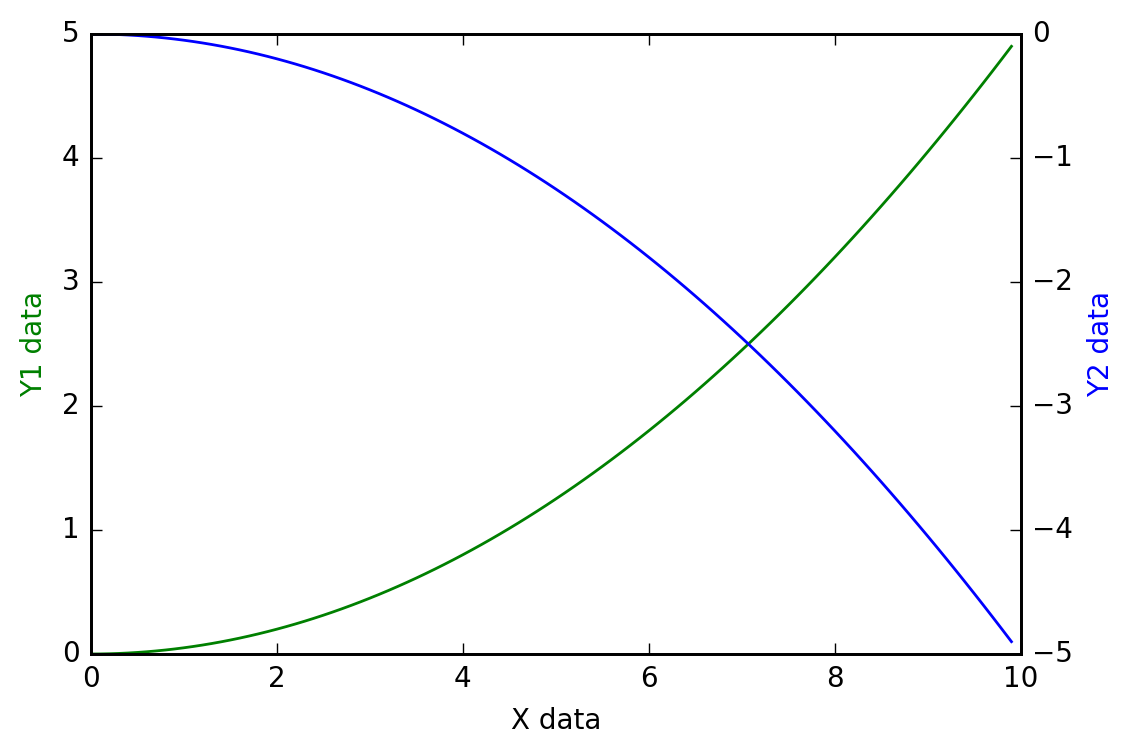# 次坐标轴

## 第一个y坐标 ¶

import matplotlib.pyplot as plt
import numpy as np

x = np.arange(0, 10, 0.1)

y1 = 0.05 * x**2

y2 = -1 * y1


fig, ax1 = plt.subplots()


## 第二个y坐标 ¶

ax1调用twinx()方法，生成如同镜面效果后的ax2

ax2 = ax1.twinx()


ax1.plot(x, y1, 'g-')   # green, solid line

ax1.set_xlabel('X data')

ax1.set_ylabel('Y1 data', color='g')

ax2.plot(x, y2, 'b-') # blue

ax2.set_ylabel('Y2 data', color='b')



plt.show()